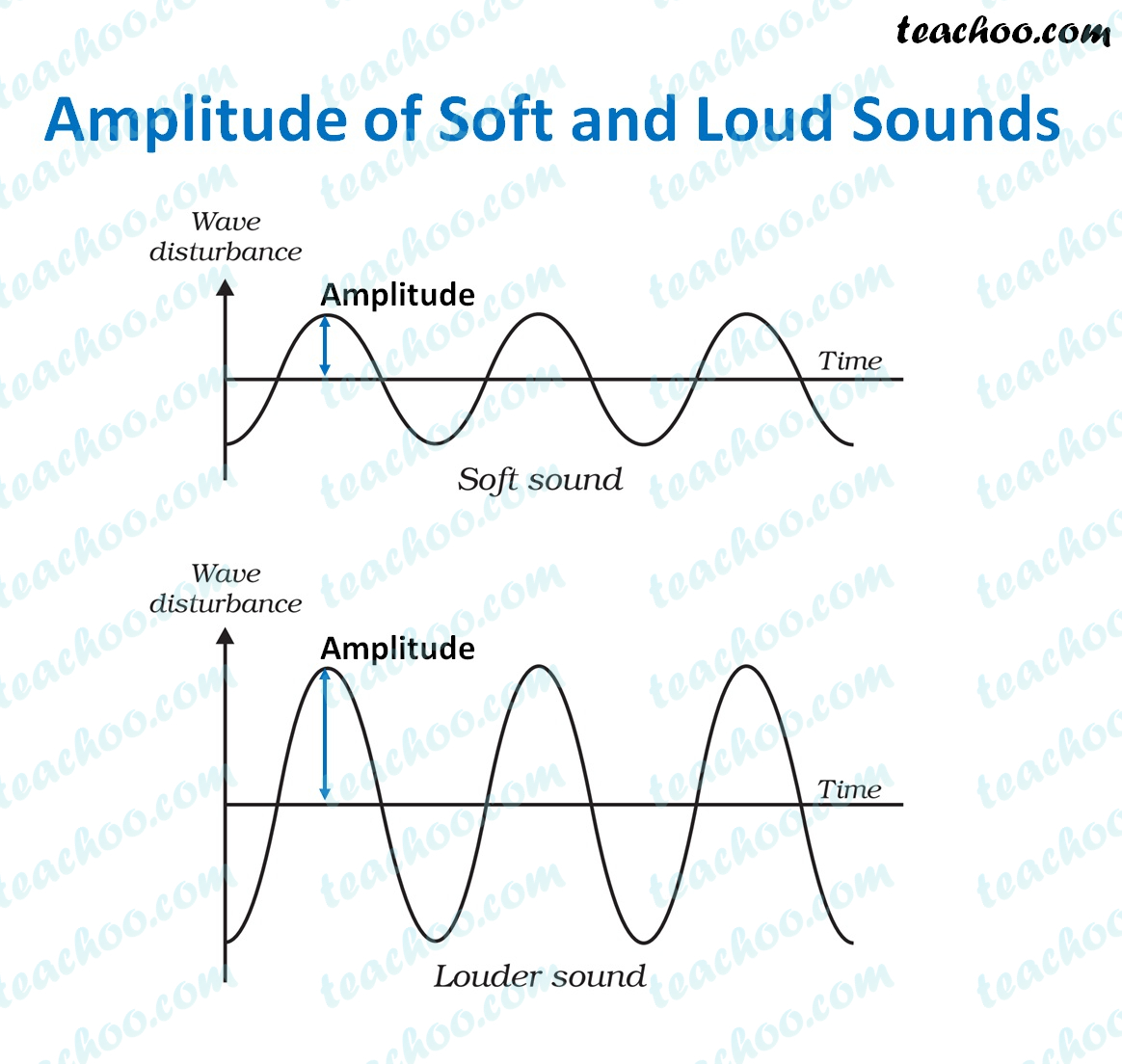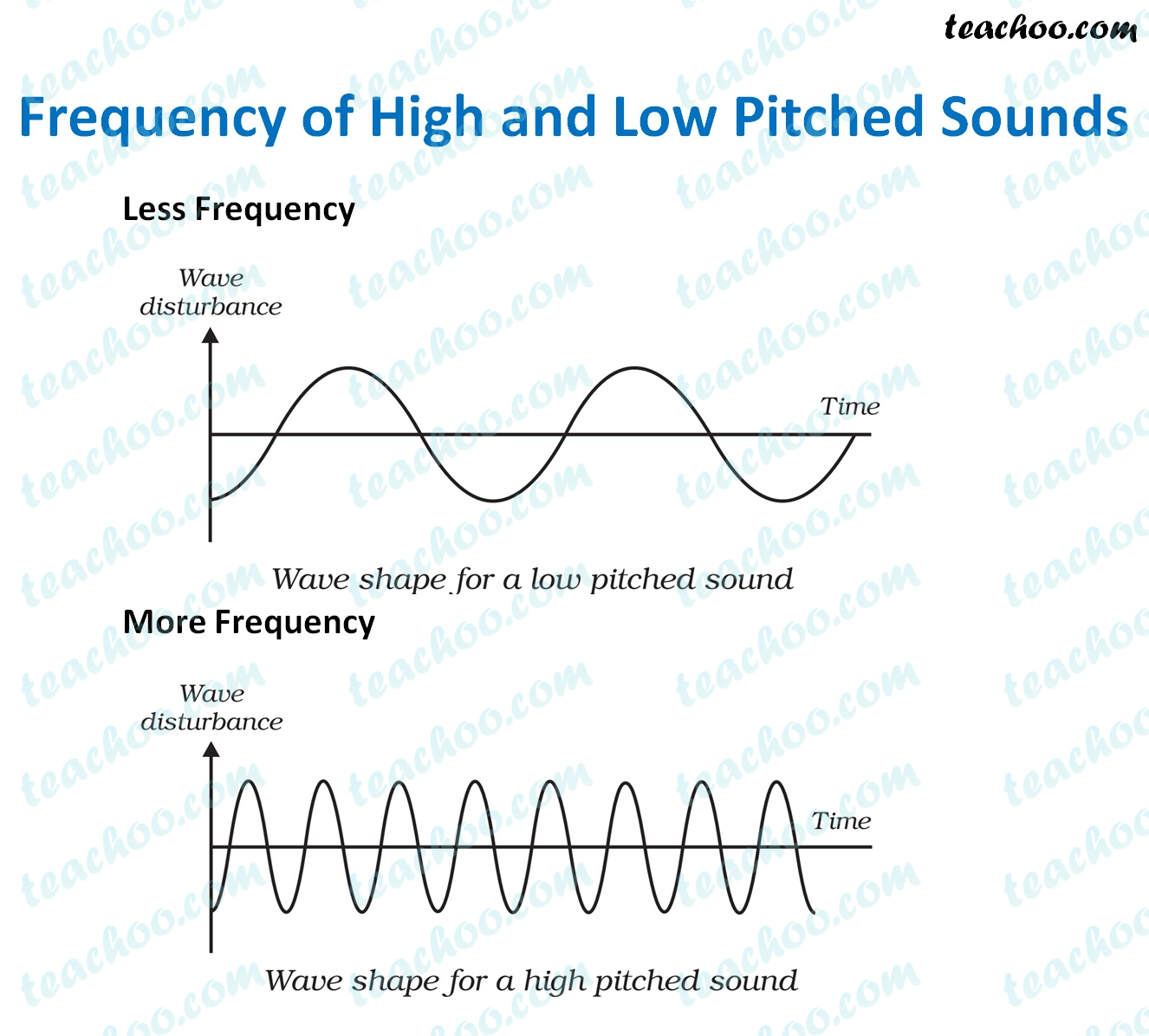Concepts

Class 8
Chapter 13 Class 8 - Sound

There are three important properties of sound

• Amplitude
• Frequency
• Time Period

## What is Amplitude

It is maximum displacement of a vibrating object from its central location

Explanation

We know that sound is produced due to vibration of particles(To and Fro Motion)

We know that in to and fro motion,an object moves from its central position

More it moves from its central position, more is the amplitude

Less it moves from its central position, less is the amplitudeNote - More Amplitude means More Loudness of Sound

Loudness is directly proportional to the square of Amplitude

If amplitude becomes double, loudness becomes 4 times

If amplitude becomes half,loudness becomes ¼ times

The loudness is expressed in a unit called decibel (dB).

Note

Roaring of lion can be heard over long distances because it has higher amplitude

## Frequency of Sound

Number of vibrations made in one second is called frequency of sound

It is measured in Hertz

Explanation

We know that sound is produced due to vibration of particles(To and Fro Motion)

More times it moves in one second,more is the frequency

Less times it moves in one second,less is frequencyExample 1

Suppose if vibration of sound is 50 vibrations per second,

it means its frequency is 50 hz

Note - Frequency determines shrillness or pitch of sound

More the frequency, more the shrillness of sound

Less the frequency, less the shrillness of sound

Example 1

Voice of Baby is of high pitch

Voice of grown up man is low pitch

Example 2

Chirping of Bird is of high pitch

Roaring of lion is of low pitch

## Time Period of Sound

The time taken by the wave to one complete oscillation is called the time period

It is measured in seconds

Relationship between Time Period and Frequency

Time Period = 1/Frequency

Example

Suppose Frequency of an object is 20 Hertz

It means that it makes 20 vibrations in 1 second

Time taken for 1 vibration=1/20=0.05 seconds

## Questions

NCERT Question 2 - Which of the following voices is likely to have minimum frequency?

Baby girl (b) Baby boy (c) A man (d) A woman

NCERT Question 3 (b) - Mark True or False

The number of oscillations per second of a vibrating object is called its time period. (T/F)

NCERT Question 3 (c) - Mark True or False

If the amplitude of vibration is large, sound is feeble. (T/F)

NCERT Question 3 (e) - Mark True or False

If the amplitude of vibration is large, sound is feeble. (T/F)

NCERT Question 4 (a) - Fill in the blanks with suitable words.

Time taken by an object to complete one oscillation is called...................

NCERT Question 4 (b) - Fill in the blanks with suitable words.

Loudness is determined by the .........................of vibration.

NCERT Question 4 (c) - Fill in the blanks with suitable words.

The unit of frequency is..........................

NCERT Question 4 (e) -  Fill in the blanks with suitable words.

Shrillness of a sound is determined by the ................. of vibration.

Learn in your speed, with individual attention - Teachoo Maths 1-on-1 Class